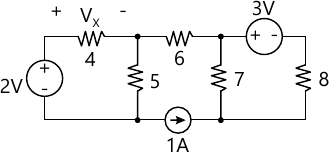MORE IN Basic Electronics
GTU First Year Engineering (Semester 2)
Basic Electronics
June 2015
Total marks: --
Total time: --
INSTRUCTIONS
(1) Assume appropriate data and state your reasons
(2) Marks are given to the right of every question
(3) Draw neat diagrams wherever necessary

Objective Question (MCQ) Choose an appropriate option from the following.
1 (a) 1 The frequency that has the longest period is
(a) 10 KHz
(b)1 KHz
(c)10 Hz
(d)1 Hz.
1 M
1 (a) 2 Ohm's law (V = IR)
(a) Can be applied to a.c similar to that of d.c.
(b)Can be applied to a.c. but after replacing R by Z (impedance)
(c)Can never be applied to a.c
(d)None of the above.
1 M
1 (a) 3 The difference in period of two frequencies of 1MHz and 2 MHz is
(a) 0.5 milli-second
(b)0.5 micro-second
(c)1 milli-second
(d)1 micro-second.
1 M
1 (a) 4 Conductance is expressed in terms of
(a) ohm / m (b) m / ohm (c) mho / m
(d)mho.
1 M
1 (a) 5 Which resistor will be physically larger in size ?
(a)10 ohm, 50 W
(b)100 ohm, 10 W
(c)1 kohm, 1 W
(d)10 Mohm, 1/ 2 W.
1 M
1 (a) 6 The output of a logic gate is '1' when all its input are at logic 0.The gate is either
(a) NAND or an EX OR gate (b) NOR or an EX-NOR gate
(c)an OR or an EX NOR gate (d) an AND or an EX-OR gate.
1 M
1 (a) 7 Out of following signals __________ is an even signal.
(a) Cosine wave
(b)Sine wave
(c)Triangle wave
(d)None of the above.
1 M
Choose an appropriate option from the following.
1 (b) 1 The circuit which magnifies a small input signal without changing its shape is called an ____________(b)Power Amplifier
(c)Non-linear Amplifier (d) None of Above.
1 M
1 (b) 2 IC741 use a ________ polarity supply.
(a) Dual
(b)Single
(c)Negative (d) None of Above.
1 M
1 (b) 3 Minimum bandwidth of an AM wave is ________.
(a) fm
(b)2fm
(c)0.5fm
(d)4fm.
1 M
1 (b) 4 PCM is a _________Pulse Modulation Technique.
(a) Analog
(b)Digital (c) Hybrid
(d)None of Above.
1 M
1 (b) 5 The first cellular systems were ___________.
(a) Analog
(b)Digital
(c)Hybrid
(d)None of Above.
1 M
1 (b) 6 A feedback control system is also called a___________.,br> (a) Recursive system (b) Linear System.
(c)Open loop system (d) Closed loop system.
1 M
1 (b) 7 CPU communicates with the outside world through the ________.
(a) Memory (b) I/O devices (c) ALU (d) None of Above.
1 M

2 (a) Define The following Terms.
(a) Interference (b) Mutual Inductance (c) Noise Margin.
3 M
2 (b) Write a short note on Cathode Ray Oscilloscope.
4 M
2 (c) Find the voltage Vx using superposition theorem. All resistor values are in ohm.7 M

3 (a) Draw circuit diagram of non-inverting operational amplifier & explain in brief.
3 M
3 (b) Explain DELTA-WYE transformation in brief with necessary equations and circuit Diagrams.
4 M
3 (c) Describe low pass active filter using Operational amplifier with necessary diagrams and equations.
7 M

4 (a) What is difference between in Microprocessor and Microcontroller?
3 M
4 (b) What do you understand about multiplexing? Explain any one of the Multiplexing Technique.
4 M
4 (c) Draw & Explain the functional description of digital communication system in Brief.
7 M

5 (a) Reduce the given function using K-map, F=Σm(1,3,5,9,11,13).
3 M
5 (b) Write Short note on D flip flop with circuit diagram and truth table.
4 M
5 (c) Classify the types of Computer network? Explain each one of them in brief.
7 M

3 M
6 (b) Classify the standard based on 2G & 3G.
4 M
6 (c) What do you understand about frequency reuse concept & Why it is used in cellular system?
7 M

7 (a) Classify the Control systems.
3 M
7 (b) Compare Open loop and Close loop System.
4 M
7 (c) Compare DSB-FC, DSB-SC, SSB, VSB.
7 M

More question papers from Basic Electronics